# How'm I doin'?

## Dollar-weighted returns give you the answer.

Paul Kaplan 7 August, 2014 | 6:00PM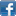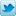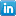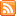Back in the late 1970s and early 1980s when I was a college student in New York City, Mayor Ed Koch would regularly ask New Yorkers, "How'm I doin'?" As investors, we often ask this question of our funds as well as of ourselves. We usually answer this question using one or more performance metrics. Some of these numbers appear prominently in reports and in fund advertising, while others must be calculated.

If you have the right data-management tool, such as Microsoft Excel, and know how to use it, you can do the calculations yourself. In this article, I review several performance metrics, explain how they are calculated, and explain which ones apply to funds and which ones to investors.

Total returns over short periods

Over a short period of time, usually a month, performance is measured using the total return for the period. The simplest way to measure the total return on a fund for a month is to add the month-end net asset value (NAV) per share to any dividends per share that the fund paid out during the month, divide the result by the NAV per share at the end of the prior month, and subtract one. For example, suppose that the NAV per share of a fund was \$10 at the end of May and that it was \$11 at the end of June and that it paid a \$1 dividend per share during June. The total return for June is (11+1)/10-1 = 0.2, or 20%. (A more precise method is to assume that the dividend is reinvested as soon as it is paid.)

Note that the total return for a month consists of two parts: (1) the percentage change in price and (2) the dividend as a percentage of the beginning price. This is because both price changes and dividends are ways in which the fund benefits investors. Of course the price change can be negative, in which case investors may suffer a loss.

Measuring fund performance

Once we've calculated the total return of a fund over a series of consecutive months, we can measure how well the fund performed over the entire period of months. To do this, we simply add 1 back to each monthly return, multiply terms, and subtract 1. For example, if the monthly total returns for April, May and June are 5%, -10% and 20% respectively, the total return for the quarter is (1.05)(0.9)(1.2)-1 = 0.134, or 13.4%.

When the period of performance is more than a year, the total return for the period is often restated as an annualized total return, also known as the compound annual return. To do this, before subtracting 1 from the product, take the nth root of the product, n being the number of years. For example, suppose that over a 60-month period the product of the monthly returns plus one was 1.47. To find the compound annual return, take the 5th root of 1.47 and subtract 1. This comes to 8%. ( See Exhibit 1, which illustrates how to make this calculation in Microsoft Excel.)

The compound annual return is the most common measure of fund performance. It appears on Morningstar fund reports for various time periods, depending on the length of the history of fund performance. It is also known as the time-weighted return.

Measuring investor performance

The only way that an investor could achieve the same return as the fund did over a given period would be to make a single investment in the fund at the beginning of the period, reinvest all dividends and make no additions or withdrawals until the very end of the period. However, investors rarely use funds in this way. Hence the returns investors earn on the funds they invest in are different from the time-weighted returns that the funds report for standard measurement periods.

The way to measure how well an investor fared is to calculate dollar-weighted returns. These returns take into account the cash that an investor adds to or withdraws from the fund holdings.

The mathematics of dollar-weighted returns are a bit complex. You cannot calculate it with a formula. Instead, you need to use a tool that solves for it. Fortunately, Microsoft Excel has such a tool in the form of a function called internal rate of return, or IRR.

To do the calculation in Excel, start by entering the initial investment in a cell. Then, below that cell, enter the series of cash flows as they occurred at regular intervals, such as once a month. Enter positive numbers for cash that you added and enter negative numbers for cash that you withdrew. Since each cell represents the cash flow for each month, enter zero if there was no cash flow in a given month. At the bottom the list, enter the final value of the investment (i.e. its latest month-end value) as a negative number.

Then use the IRR function with the entire range of cells, from the initial investment through the negative of the final value, as the argument to the function. If the cash flows are monthly, this will give the dollar-weighted return as a monthly number. To convert to an annual rate of return, add 1, raise to the 12th power, and subtract one. ( Exhibit 2 shows how to use the IRR function and convert the result to an annual return.)

The dollar-weighted return is the single rate of return that, if applied to the initial investment and all cash flows, would result in the final value of the investment. It depends not only on the performance of funds that the investor holds, but also the timing of the cash flows.

For example, imagine that you invest \$1,000 in a fund and add \$1,000 a month for 10 years. The initial \$1,000 is invested for 120 months, but each subsequent \$1,000 is invested for one month less than the prior \$1,000 until you get the final \$1,000, which is only invested for one month. Hence each monthly total return on the fund affects a different amount of money. In contrast, each monthly return affects the time-weighted return in the same way.

To see how time-weighted and dollar-weighted returns can differ, I calculated both of these returns on a set of indexes relevant to Canadian investors over the 10-year period from July 2004 to June 2014. I calculated the dollar-weighted returns making the assumption that I discussed above -- that \$1,000 is invested at the beginning of each month. As Exhibit 3 shows, time-weighted and dollar-weighted returns generally differ and sometimes by a significant amount.

To sum up

Time-weighted and dollar-weighted returns are both important performance metrics. Time-weighted returns are appropriate for measuring how well fund managers did over a given time period. However, how well fund investors did not only depends on fund returns, but also in the timing of their investments in the fund. Dollar-weighted returns are the appropriate way to measure this.•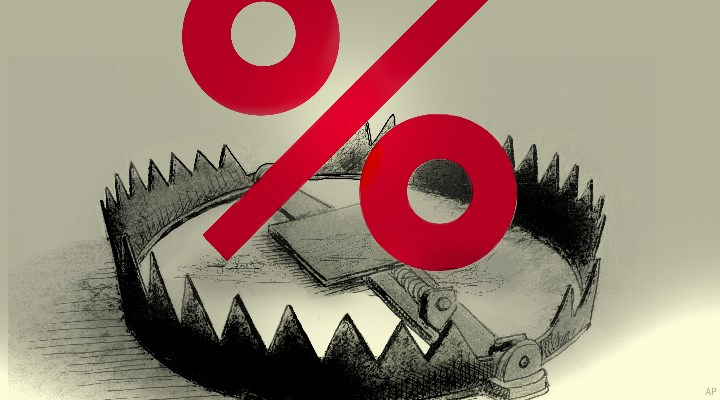4 Canadian High-Yield Dividend Stocks to Avoid

These stocks have juicy yields – but these dividends might not be sustainable.

•How Much Cash Should You Have on Hand Today?

Enough for an emergency fund, but some investors should set aside even more cash. Here's why.

•Twitter Nosedives After Musk Pumps Brakes on Deal

Twitter shares, which have been trading at a discount to Elon Musk's offer amid skepticism the de...

•Why is National Bank so Cheap?

Great Canadian exposure with less of the overpriced housing.

•Five Common Scams (And The Blindspots That Make You Vulnerable)

From a desire to get things done to misplaced confidence in internet security, scammers are thriv...